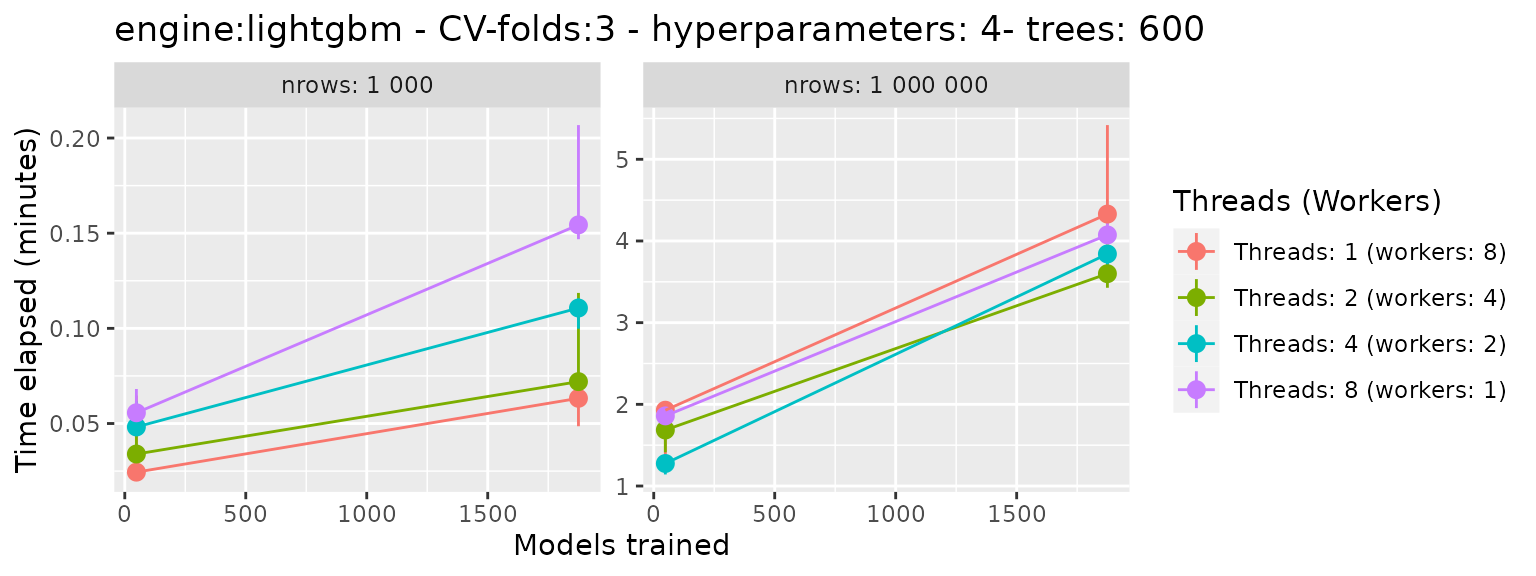## Findings:

• Forking better for small datasets
• Combination of Forking and Threading is better for large datasets## Parameters (all combinations of…):

• the size of the data.frame: 1.000 vs 1.000.000 rows
• the number of models trained in grid search: 48 vs 1875
• threads/workers: 1/8, 2/4, 4/2 and 8/1 (there was a constraint of workers*threads = 8)

## Machine:

• Intel Core i7-8565U
• CPU @ 1.80GHz × 8 cores
• Dell Inspiron
• RAM 16GB
• Linux Ubuntu 20.04 64bit

## Code:

library(tidymodels)
library(treesnip)
# main function to tune_grid with given benchmark parameters
execute_tune_grid <- function(df, threads, workers, cv_folds, tune_grid, engine = "lightgbm") {
##############################################################
df_splits <- vfold_cv(df, v = cv_folds)
df_rec <- recipe(mpg ~ ., data = df)
df_model <- boost_tree(
mtry = 3,
trees = 600,
sample_size = 0.7,
min_n = tune(),
tree_depth = tune(),
learn_rate = tune(),
loss_reduction = tune()
) %>%
set_mode("regression") %>%

df_wf <- workflow() %>%

##############################################################
doParallel::registerDoParallel(workers)
tg <- tune_grid(df_wf, df_splits, grid = tune_grid)

tg
}

# parameters
parameters <- expand.grid(
engine = "lightgbm",
cv_folds = 3,
tune_grid = c(2, 5),
nrow = c(1e3, 1e6),
threads = c(1, 2, 4, 8)
) %>%
mutate(
)

# bench::mark
set.seed(1)
bm <- bench::press(
{
Sys.sleep(3)
df <- mtcars %>% sample_n(nrow, replace = TRUE)
bench::mark(
execute_tune_grid(df, threads, workers, cv_folds, tune_grid, engine),
check = FALSE,
iterations = 3,
memory = FALSE,
filter_gc = FALSE,
time_unit = "m"
)
},
.grid = parameters
)